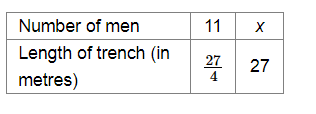# How many men should be employed for digging 27-metre-long trench of the same type in one day?Question:

11 men can dig $6 \frac{3}{4}$-metre-long trench in one day. How many men should be employed for digging 27-metre-long trench of the same type in one day?

Solution:

Let $x$ be the required number of men.

Now, $6 \frac{3}{4} \mathrm{~m}=\frac{27}{4} \mathrm{~m}$

Then, we have:Clearly, the longer the trench, the greater will be the number of men required.

So, it is a case of direct proportion.

Now, $\frac{11}{\frac{27}{4}}=\frac{x}{27}$

$\Rightarrow \frac{11 \times 4}{27}=\frac{x}{27}$

$\Rightarrow x=44$

Therefore, 44 men should be employed to dig a trench of length 27 m.